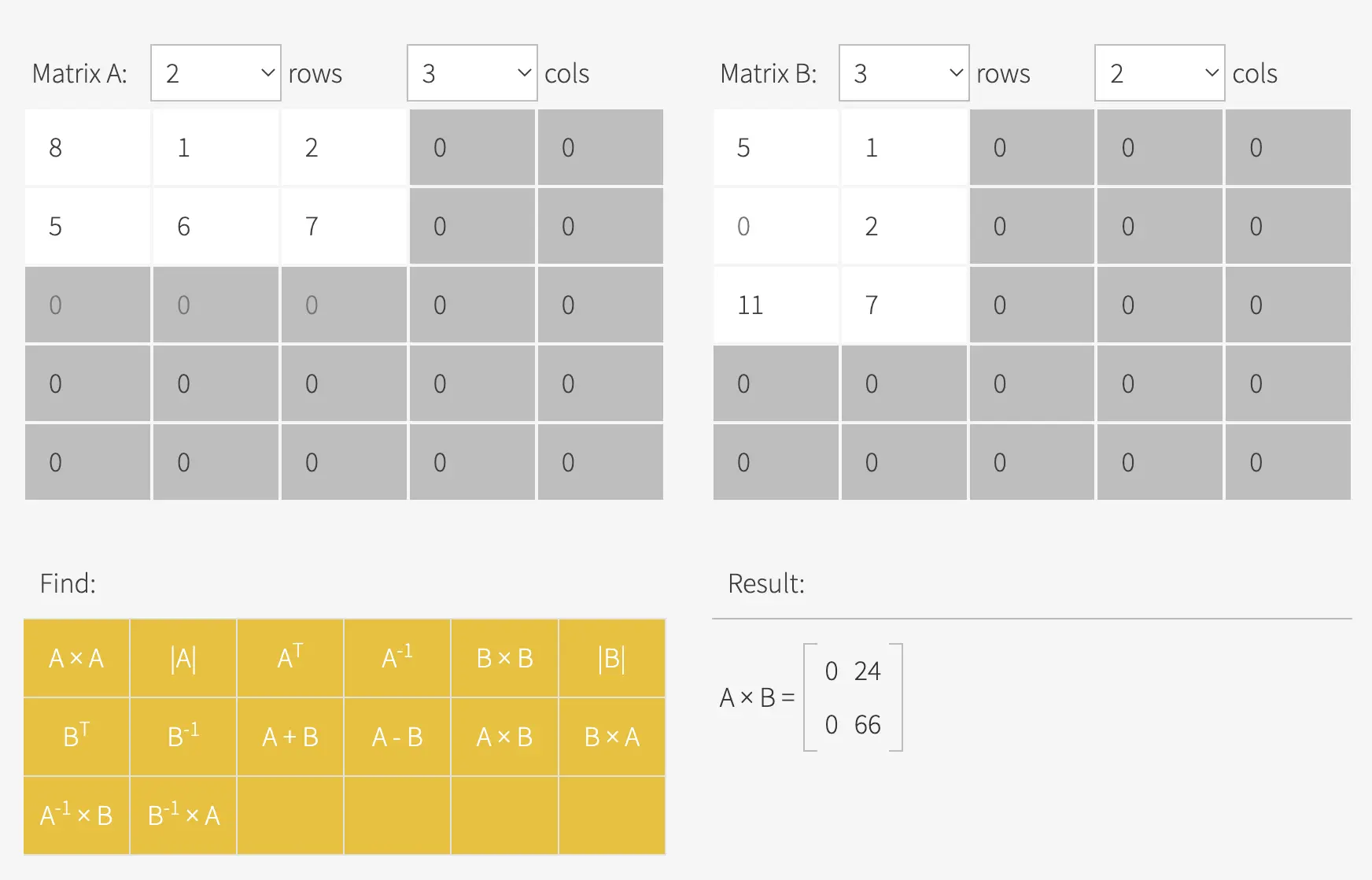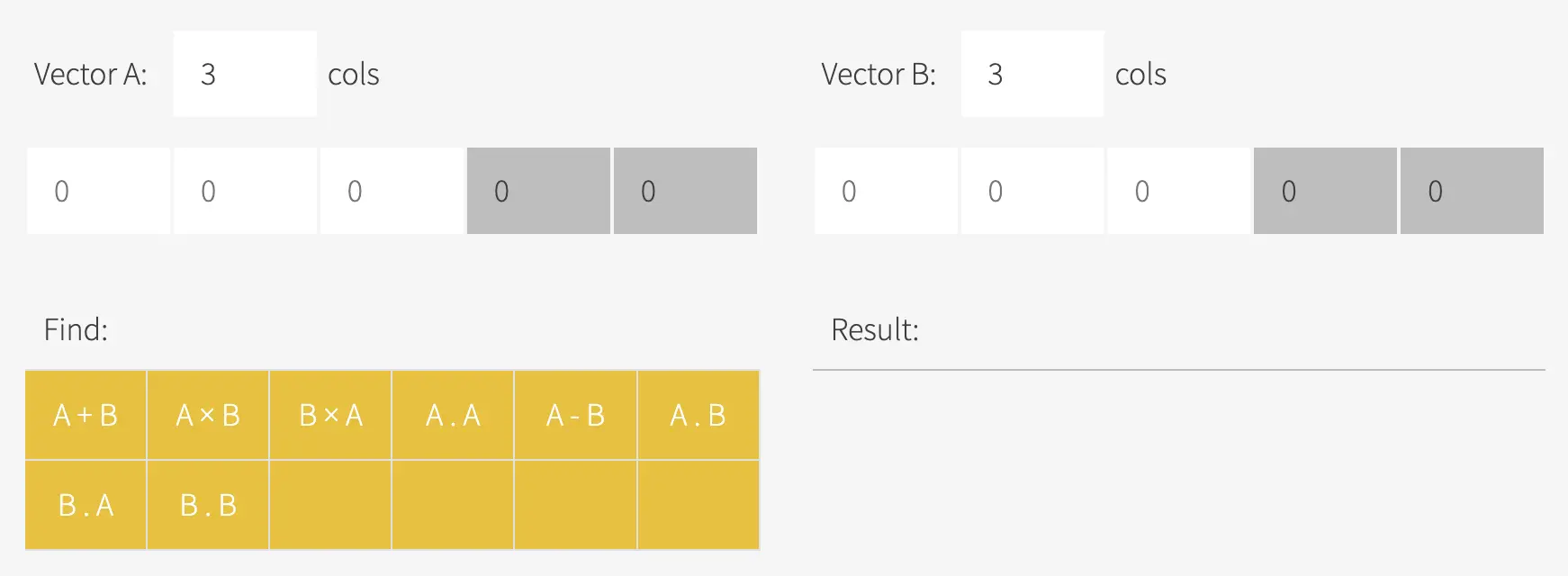# Matrix / Vector Calculator

• Our matrix and vector calculator is the most sophisticated and comprehensive matrix calculator online.
• You can add, subtract, multiply and transpose matrices. Easily perform matrix multiplication with complex numbers.
• Calculate the determinant or inverse of a matrix.# Best Matrix Calculator Online

Matrix Calculator determines the resultant matrix of two given matrices assigned with arithmetic operations.

In mathematical concepts, a matrix is a rectangular array consisting of numbers, symbols, or expressions arranged in rows and columns, used to demonstrate an object's property or a mathematical object.

Matrix formulas have been used to solve calculus and linear equations, quantum mechanics, optics, and other mathematical operations.

Matrices can be solved through the following arithmetic operations:

1. Addition of matrices - Two matrices can be added if they have the same number of rows and columns. For this operation, just add the elements in corresponding positions.
2. Subtraction of matrices - Two matrices can be subtracted if they have the same number of rows and columns. Subtract the elements of each row and column of the second matrix from the respective elements of the first matrix.
3. Multiplication of matrices - In multiplication, the number of rows in one matrix should be equal to the number of columns in the previous matrix.

Our comprehensive online matrix calculator will let you add, subtract, multiply, and transpose matrices. You can also find the determinant or inverse of a matrix using our matrix calculator.

Here’s an example of multiplying matrices using our online matrix calculator:Figure 1. Example of matrix multiplicationFigure 2. Screenshot of the vector calculator